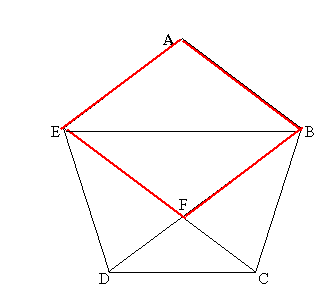#### You may also like### Golden Thoughts

Rectangle PQRS has X and Y on the edges. Triangles PQY, YRX and XSP have equal areas. Prove X and Y divide the sides of PQRS in the golden ratio.### At a Glance

The area of a regular pentagon looks about twice as a big as the pentangle star drawn within it. Is it?### Areas and Ratios

Do you have enough information to work out the area of the shaded quadrilateral?

# Pent

##### Age 14 to 18Challenge LevelThe diagram shows a regular pentagon with sides of unit length. Find all the angles in the diagram. Prove that the quadrilateral shown in red is a rhombus. Prove that triangles EFB and DFC are similar and hence show that the length EB is equal to the golden ratio and find the ratio BF : FD.